# RS Aggarwal Class 7 Solutions Chapter 2 Fractions CCE Test Paper

## RS Aggarwal Class 7 Solutions Chapter 2 Fractions CCE Test Paper

These Solutions are part of RS Aggarwal Solutions Class 7. Here we have given RS Aggarwal Solutions Class 7 Chapter 2 Fractions CCE Test Paper.

Other Exercises

Question 1.
Solution:
(i) A number of the form $$\frac { a }{ b }$$, where a and b are rational numbers, is called a natural number.
Here, a is the numerator and b is the denominator.
(a) $$\frac { 2 }{ 3 }$$ is a fraction with 2 as the numerator and 3 as the denominator.
(b) $$\frac { 12 }{ 5 }$$ is a fraction with 12 as the numerator and 5 as the denominator.
(ii) A fraction whose denominator is a whole number other than 10, 100, 1000, etc., is called a vulgar faction.
Examples : $$\frac { 2 }{ 5 }$$ and $$\frac { 4 }{ 15 }$$.
(iii) A fraction whose numerator is greater than or equal to its denominator is called an improper fraction.
Example : $$\frac { 11 }{ 3 }$$ and $$\frac { 41 }{ 35 }$$

Question 2.
Solution: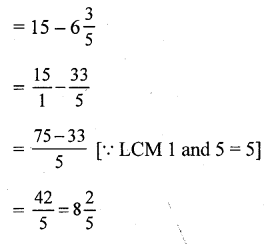Hence, the required number is 8$$\frac { 2 }{ 5 }$$

Question 3.
Solution: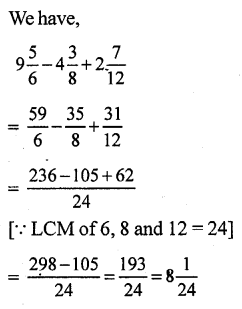Question 4.
Solution: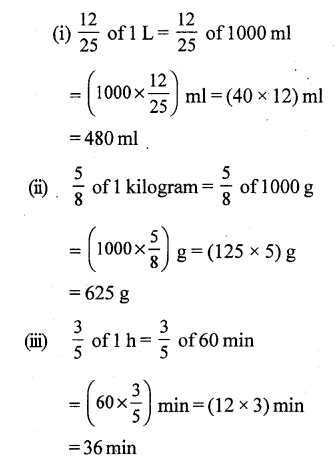Question 5.
Solution: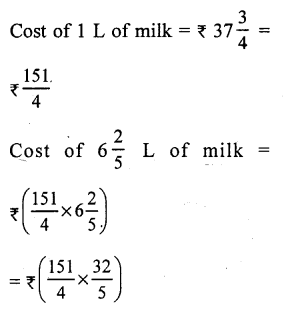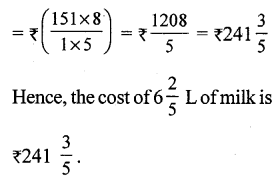Question 6.
Solution: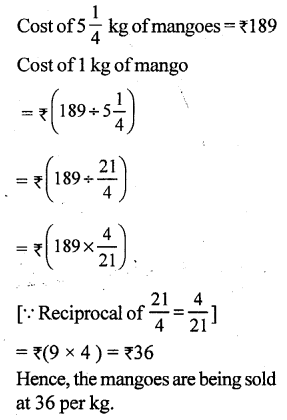Question 7.
Solution: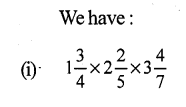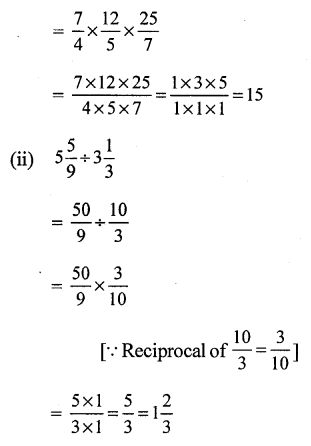Question 8.
Solution: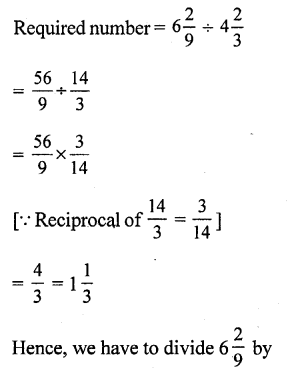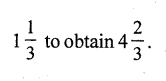Question 9.
Solution: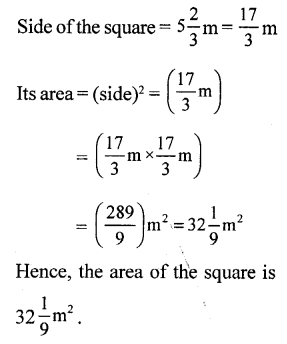Mark (√) against the correct answer in each of the following:

Question 10.

Solution:
(d) $$\frac { 5 }{ 8 }$$
$$\frac { 5 }{ 8 }$$ is a vulgar fraction, because its denominator is other than 10,100, 1000, etc.

Question 11.
Solution:
(c) $$\frac { 46 }{ 63 }$$
A fraction $$\frac { a }{ b }$$ is said to be irreducible or in its lowest terms if the HCF of a and b is 1
46 = 2 x 23 x 1
63 = 3 x 3 x 21 x 1
Clearly, the HCF of 46 and 63 is 1.
Hence, $$\frac { 46 }{ 63 }$$ is an irreducible fraction.

Question 12.
Solution:
(d) None of these
Reciprocal of 1$$\frac { 3 }{ 5 }$$ = Reciprocal of $$\frac { 8 }{ 5 }$$ = $$\frac { 5 }{ 8 }$$

Question 13.
Solution: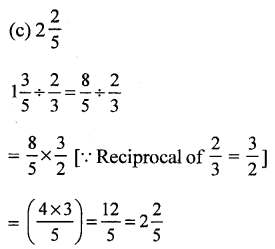Question 14.
Solution: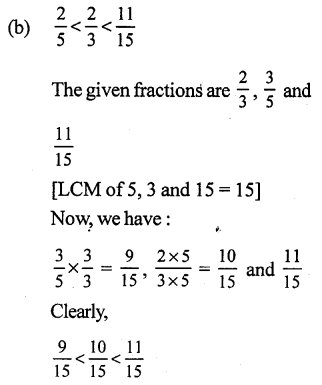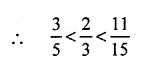Question 15.
Solution: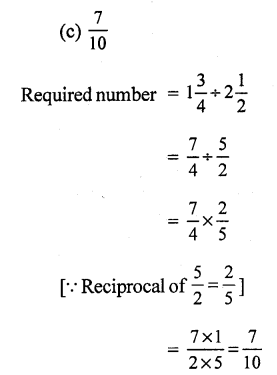Question 16.
Solution:
(b) 33 km
Distance covered by the car on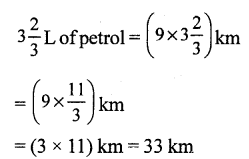Question 17.
Solution: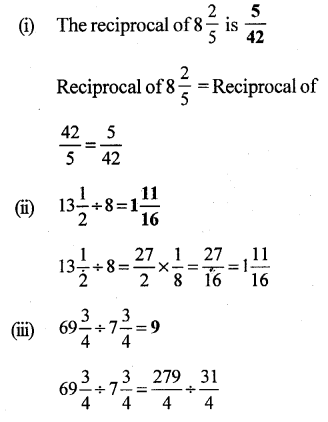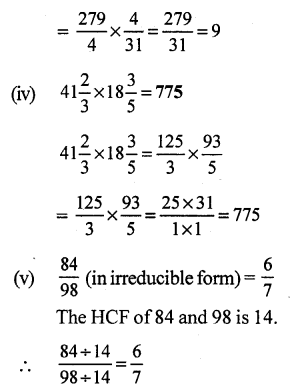Question 18.
Solution:
(i) False.
By cross multiplication, we have:
9 x 24 = 216 and 13 x 16 = 208
However, 216 > 208
$$\frac { 9 }{ 16 }$$ > $$\frac { 13 }{ 24 }$$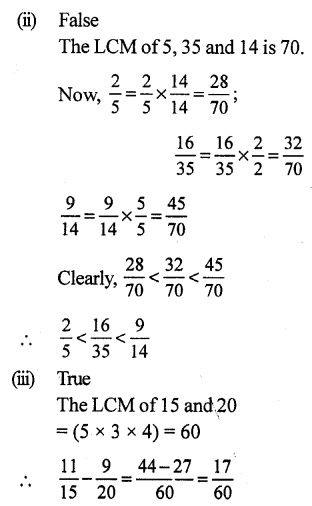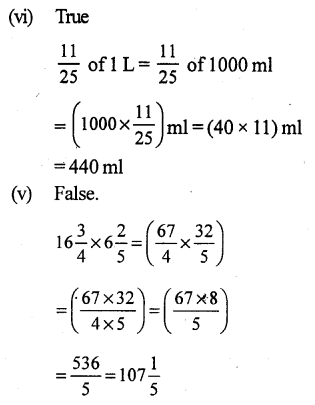Hope given RS Aggarwal Solutions Class 7 Chapter 2 Fractions CCE Test Paper are helpful to complete your math homework.

If you have any doubts, please comment below. Learn Insta try to provide online math tutoring for you.